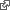#Method Expressions

Method gets and sets variables with long wordy keys. Here we use the context of a single Method to interpret an expression where values can be named by a small but still unique fragment of already retrieved keys.

The existing middle-school math encourages step by step calculations that are hard to misinterpret. Mostly this is due to long and more precise names.

Say CALC to calculate a formula that appears on the remainder of the line.

The formula, without the word CALC, becomes the name of the result and can be used to recall it in later calcuations.

### Example

Consider computing a paycheck with time and a half for overtime.

16.45 Hourly Rate 40 Regular Hours PRODUCT Base Pay

Hourly Rate 12 Overtime Hours 1.5 Overtime Premium PRODUCT Overtime Pay Base Pay SUM Total Pay

This could be more concisely expressed using a formula.

16.45 Hourly Rate 40 Regular Hours 12 Overtime Hours CALC Rate * ( Regular + 1.5 * Overtime )

The challenge here is to define CALC to use the label field as a formula written in terms of numbers already defined in the Method. Values are more conveniently identified by single words. Here we will use a partial match on extant value names to give these words meaning.

The formula itself will become the name of the result. However, one might reasonably offer a more descriptive name in a subsequent line, perhaps a SHOW operation.

The formula works with the units present in other values and will return a result with appropriate units.

16.45 Rate (dollar / hour) 40 Regular (hour) 12 Overtime (hour) CALC Rate * ( Regular + 1.5 * Overtime )

Hover over the results from this and the previous calculation to compare the difference that units makes.

### Resources

Douglas Crockfordjavascript evaluator using Pratt's top-down operator precedence.

Jxcksimple recursive-descent evaluator in a 90 line gist including tests.

About Method Plugin for regular documentation.